## Delta Function Potentials in One Dimension

The delta function potential is a very useful one to make simple models of molecules and solids. First we solve the problem with one attractive delta function. Since the bound state has negative energy, the solutions that are normalizable arefor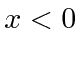andfor. Making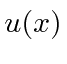continuous and its first derivative have a discontinuity computed from the Schrdinger equation at, gives us exactly one bound state with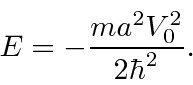Next we use two delta functions to model a molecule,. Solving this problem by matching wave functions at the boundaries at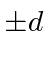, we find again transcendental equations for two bound state energies. The ground state energy is more negative than that for one delta function, indicating that the molecule would be bound. A look at the wavefunction shows that the 2 delta function state can lower the kinetic energy compared to the state for one delta function, by reducing the curvature of the wavefunction. The excited state has more curvature than the atomic state so we would not expect molecular binding in that state.

Our final 1D potential, is a model of a solid.This has a infinite, periodic array of delta functions, so this might be applicable to a crystal. The solution to this is a bit tricky but it comes down to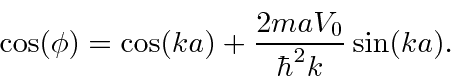Since the right hand side of the equation can be bigger than 1.0 (or less than -1), there are regions ofwhich do not have solutions. There are also bands of energies with solutions. These energy bands are seen in crystals (like Si).

Jim Branson 2013-04-22